# Chapter 14 Co-Ordinate Geometry RD Sharma Solutions Exercise 14.4 Class 10 Maths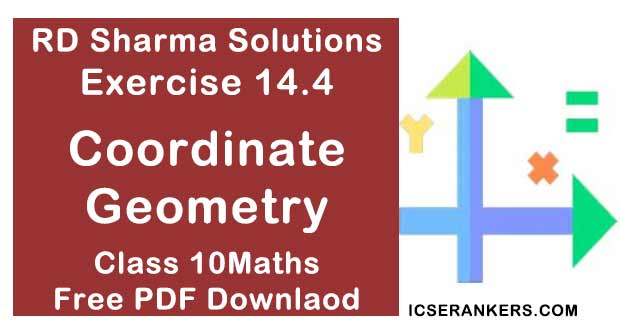Chapter Name RD Sharma Chapter 14 Co-Ordinate Geometry Book Name RD Sharma Mathematics for Class 10 Other Exercises Exercise 14.1Exercise 14.2Exercise 14.3Exercise 14.5 Related Study NCERT Solutions for Class 10 Maths

### Exercise 14.4 Solutions

1. Find the centroid of the triangle whose vertices are :
(i) (1, 4), (-1, -1) and (3, -2)

Solution

We know that the coordinates of the centroid of a triangle whose vertices are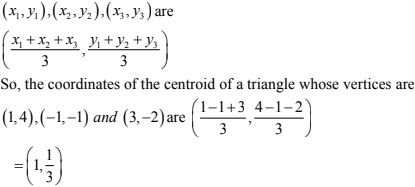2. Two vertices of a triangle are (1, 2), (3, 5) and its centroid is at the origin. Find the coordinates of the third vertex.

Solution

Let the coordinates of the third vertex bee (x, y), Then
Coordinates of centroid of triangle are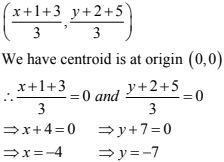3. Prove analytically that the line segment joining the middle points of two sides of a triangle is equal to half of the third side.

Solution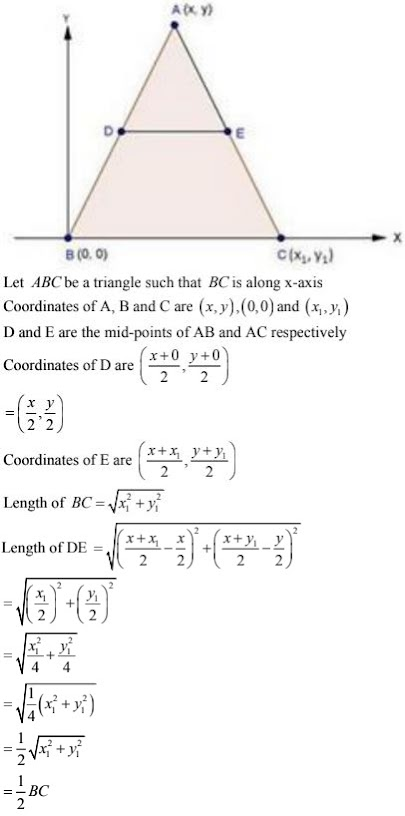4. Prove that the lines joining the middle points of the opposite sides of a quadrilateral and the join of the middle points of its diagonals meet in a point and bisect one another.

Solution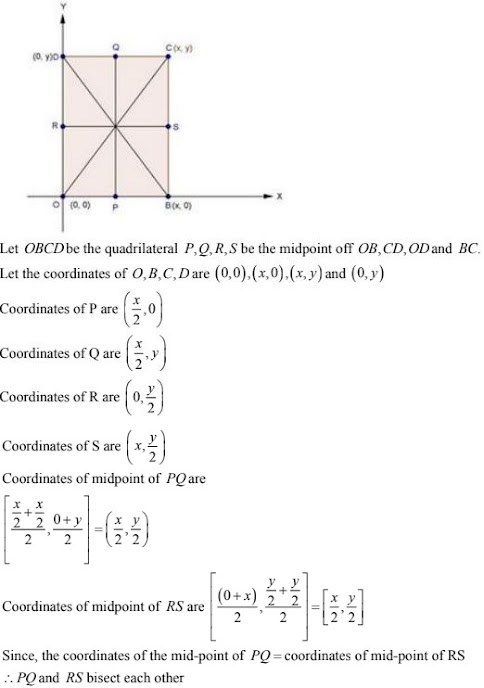5. If G be the centroid of a triangle ABC and P be any other point in the plane, prove that PA2 + PB2 + PC2 = GA2 + GB2 + GC2 + 3GP2 .

Solution6. If G be the centroid of a triangle ABC, prove that :
AB2 + BC2 + CA2 = 3(GA2 + GB2 + GC2)
Solution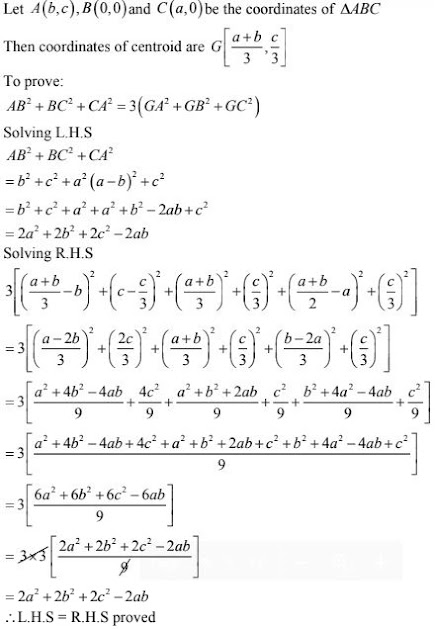7. If (-2, 3), (4, -3) and (4, 5) are the mid - points of the sides of a triangle, find the coordinates of its centroid.
Solution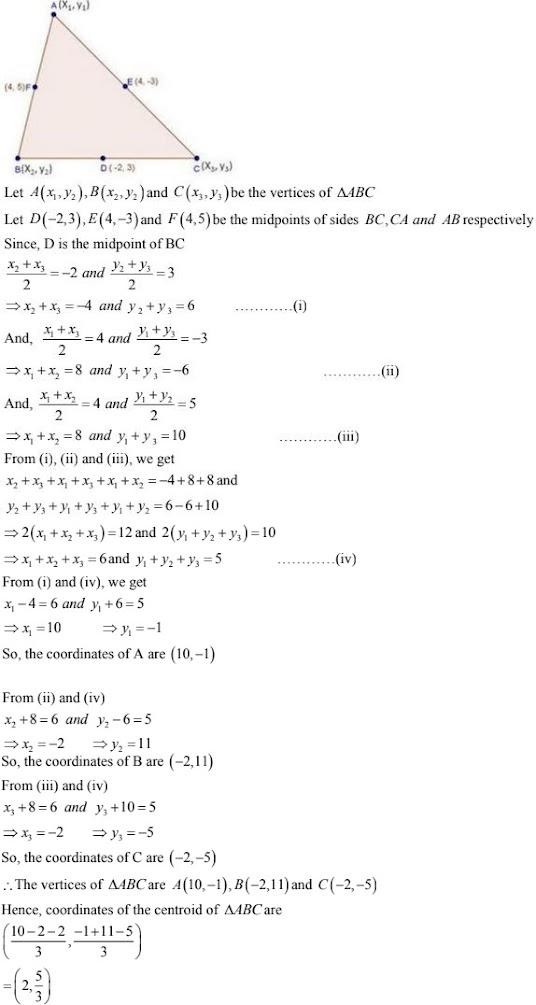8. In below Fig. a right triangle BOA is given. C is the mid-point of the hypotenuse AB. Show that it is equidistant from the vertices O, A and B.

Solution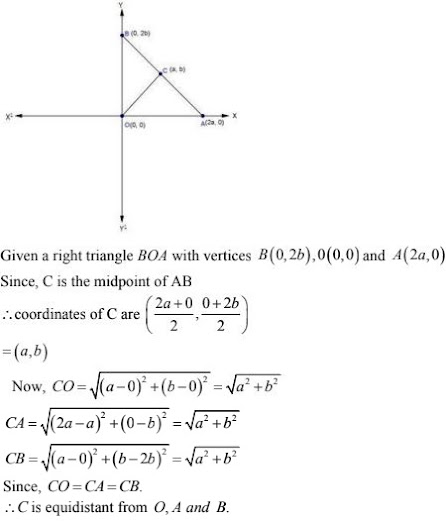9. Find the third vertex of a triangle, if two of tis vertices are at (-3, 1) and (0, -2) and the centroid is at the origin.

Solution

Let the coordinates of the third vertex be (x, y), Then
Coordinates of centroid of triangle are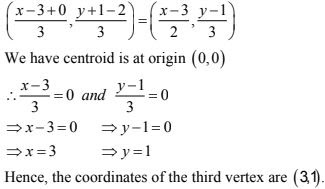10. A(3, 2) and (-2, 1) are two vertices of a triangle ABC whose centroid G has the coordinates (5/11, 1/3). Find the coordinates of the third vertex C of the triangle.

Solution

Let the third vertex be C(x, y)
Two vertices A(3, 2) and B(-2, 1)
Coordinate of centroid of triangle are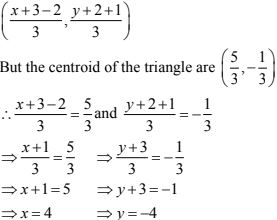Hence, the third vertex of the triangle is C(4, -4).